# The FREQ Procedure

### OUTPUT Statement

• OUTPUT < OUT=SAS-data-set > output-options;

The OUTPUT statement creates a SAS data set that contains statistics that are computed by PROC FREQ. Table 40.7 lists the statistics that can be stored in the output data set. You identify which statistics to include by specifying output-options.

You must use a TABLES statement with the OUTPUT statement. The OUTPUT statement stores statistics for only one table request. If you use multiple TABLES statements, the contents of the output data set correspond to the last TABLES statement. If you use multiple table requests in a single TABLES statement, the contents of the output data set correspond to the last table request. Only one OUTPUT statement is allowed in a single invocation of the procedure.

For a one-way or two-way table, the output data set contains one observation that stores the requested statistics for the table. For a multiway table, the output data set contains an observation for each two-way table (stratum) of the multiway crosstabulation. If you request summary statistics for the multiway table, the output data set also contains an observation that stores the across-strata summary statistics. If you use a BY statement, the output data set contains an observation or set of observations for each BY group. For more information about the contents of the output data set, see the section Contents of the OUTPUT Statement Output Data Set.

The output data set that is created by the OUTPUT statement is not the same as the output data set that is created by the OUT= option in the TABLES statement. The OUTPUT statement creates a data set that contains statistics (such as the Pearson chi-square and its p-value), and the OUT= option in the TABLES statement creates a data set that contains frequency table counts and percentages. See the section Output Data Sets for more information.

As an alternative to the OUTPUT statement, you can use the Output Delivery System (ODS) to store statistics that PROC FREQ computes. ODS can create a SAS data set from any table that PROC FREQ produces. See the section ODS Table Names for more information.

You can specify the following options in the OUTPUT statement:

OUT=SAS-data-set

specifies the name of the output data set. When you use an OUTPUT statement but do not use the OUT= option, PROC FREQ creates a data set and names it by using the DATAn convention.

output-options

specify the statistics to include in the output data set. Table 40.7 lists the output-options that are available in the OUTPUT statement, together with the TABLES statement options that are required to produce the statistics. Descriptions of the output-options follow the table in alphabetical order.

You can specify output-options to request individual statistics, or you can request groups of statistics by using output-options that are identical to the group options in the TABLES statement (for example, the CHISQ , MEASURES , CMH , AGREE , and ALL options).

When you specify an output-option, the output data set includes statistics from the corresponding analysis. In addition to the estimate or test statistic, the output data set includes associated values such as standard errors, confidence limits, p-values, and degrees of freedom. For more information, see the section Contents of the OUTPUT Statement Output Data Set.

To store a statistic in the output data set, you must also request computation of that statistic with the appropriate TABLES , EXACT , or TEST statement option. For example, the PCHI output-option includes the Pearson chi-square in the output data set. You must also request computation of the Pearson chi-square by specifying the CHISQ option in the TABLES statement. Or, if you use only one TABLES statement, you can request computation of the Pearson chi-square by specifying the PCHI or CHISQ option in the EXACT statement. Table 40.7 lists the TABLES statement options that are required to produce the OUTPUT data set statistics.

Table 40.7: OUTPUT Statement Output Options

Output Option

Output Data Set Statistics

Required TABLES Statement Option

McNemar’s test (tables), Bowker’s test,

simple and weighted kappas; for multiple strata,

overall simple and weighted kappas, tests for equal

kappas, and Cochran’s Q (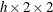tables)

Continuity-adjusted chi-square (tables)

CHISQ , MEASURES , and CMH statistics;

N (number of nonmissing observations)

Breslow-Day test (tables)

CMH , CMH1 , or CMH2

Binomial statistics (one-way tables)

For one-way tables, goodness-of-fit test;

for two-way tables, Pearson, likelihood ratio,

chi-squares, Fisher’s exact test (tables),

phi and contingency coefficients, Cramér’s V

Cochran-Mantel-Haenszel (CMH) correlation,

row mean scores (ANOVA), and general

association statistics; fortables, logit

and Mantel-Haenszel common odds ratios

and relative risks, Breslow-Day test

CMH statistics, except row mean scores (ANOVA)

CMH or CMH1

and general association statistics

CMH statistics, except general association statistic

CMH or CMH2

CMH correlation statistic

CMH , CMH1 , or CMH2

CMH general association statistic

CMH row mean scores (ANOVA) statistic

CMH or CMH2

Cochran’s Q (tables)

Contingency coefficient

Cramér’s V

Test for equal simple kappas

Zelen’s test for equal odds ratios (tables)

Test for equal weighted kappas

Fisher’s exact test

Gamma

Gail-Simon test

Jonckheere-Terpstra test

Simple kappa coefficient

Kendall’s tau-b

Lambda asymmetric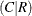Lambda symmetric

Lambda asymmetric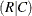Logit common odds ratio

CMH , CMH1 , or CMH2

Logit common relative risk, column 1

CMH , CMH1 , or CMH2

Logit common relative risk, column 2

CMH , CMH1 , or CMH2

Likelihood ratio chi-square

McNemar’s test (tables)

Gamma, Kendall’s tau-b, Stuart’s tau-c,

Somers’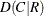and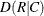, Pearson and

Spearman correlations, lambda asymmetricand, lambda symmetric,

uncertainty coefficientsand,

symmetric uncertainty coefficient;

odds ratio and relative risks (tables)

Mantel-Haenszel chi-square

Mantel-Haenszel common odds ratio

CMH , CMH1 , or CMH2

Mantel-Haenszel common relative risk, column 1

CMH , CMH1 , or CMH2

Mantel-Haenszel common relative risk, column 2

CMH , CMH1 , or CMH2

Number of nonmissing observations

Number of missing observations

Odds ratio (tables)

or RELRISK

Chi-square goodness-of-fit test (one-way tables),

Pearson chi-square (two-way tables)

Pearson correlation coefficient

Phi coefficient

Polychoric correlation coefficient

Column 1 risk difference (row 1 – row 2)

Column 2 risk difference (row 1 – row 2)

Odds ratio and relative risks (tables)

Risks and risk differences (tables)

Risks and risk difference, column 1

Risks and risk difference, column 2

Relative risk, column 1

Relative risk, column 2

Column 1 overall risk

Column 1 risk for row 1

Column 2 risk for row 1

Column 2 overall risk

Column 1 risk for row 2

Column 2 risk for row 2

Spearman correlation coefficient

Somers’Somers’Stuart’s tau-c

Cochran-Armitage test for trend

Bowker’s test of symmetry

Symmetric uncertainty coefficient

Uncertainty coefficientUncertainty coefficientWeighted kappa coefficient

[a] CHISQ computes Fisher’s exact test fortables. Use the FISHER option to compute Fisher’s exact test for generaltables.

You can specify the following output-options in the OUTPUT statement.

AGREE

includes the following tests and measures of agreement in the output data set: McNemar’s test (fortables), Bowker’s test of symmetry, the simple kappa coefficient, and the weighted kappa coefficient. For multiway tables, the AGREE option also includes the following statistics in the output data set: overall simple and weighted kappa coefficients, tests for equal simple and weighted kappa coefficients, and Cochran’s Q test.

The AGREE option in the TABLES statement requests computation of tests and measures of agreement. For more information, see the section Tests and Measures of Agreement.

AGREE statistics are computed only for square tables, where the number of rows equals the number of columns. PROC FREQ provides Bowker’s test of symmetry and weighted kappa coefficients only for tables larger than. (Fortables, Bowker’s test is identical to McNemar’s test, and the weighted kappa coefficient equals the simple kappa coefficient.) Cochran’s Q is available for multiwaytables.

AJCHI

includes the continuity-adjusted chi-square in the output data set. The continuity-adjusted chi-square is available fortables and is provided by the CHISQ option in the TABLES statement. For more information, see the section Continuity-Adjusted Chi-Square Test.

ALL

includes all statistics that are requested by the CHISQ , MEASURES , and CMH output-options in the output data set. ALL also includes the number of nonmissing observations, which you can request individually by specifying the N output-option.

BDCHI

includes the Breslow-Day test in the output data set. The Breslow-Day test for homogeneity of odds ratios is computed for multiwaytables and is provided by the CMH , CMH1 , and CMH2 options in the TABLES statement. For more information, see the section Breslow-Day Test for Homogeneity of the Odds Ratios.

BINOMIAL
BIN

includes the binomial proportion estimate, confidence limits, and tests in the output data set. The BINOMIAL option in the TABLES statement requests computation of binomial statistics, which are available for one-way tables. For more information, see the section Binomial Proportion.

CHISQ

includes the following chi-square tests and measures in the output data set for two-way tables: Pearson chi-square, likelihood ratio chi-square, Mantel-Haenszel chi-square, phi coefficient, contingency coefficient, and Cramér’s V. Fortables, CHISQ also includes Fisher’s exact test and the continuity-adjusted chi-square in the output data set. For more information, see the section Chi-Square Tests and Statistics. For one-way tables, CHISQ includes the chi-square goodness-of-fit test in the output data set. For more information, see the section Chi-Square Test for One-Way Tables. The CHISQ option in the TABLES statement requests computation of these statistics.

If you specify the CHISQ(WARN=OUTPUT) option in the TABLES statement, the CHISQ option also includes the variable WARN_PCHI in the output data set. This variable indicates the validity warning for the asymptotic Pearson chi-square test.

CMH

includes the following Cochran-Mantel-Haenszel statistics in the output data set: correlation, row mean scores (ANOVA), and general association. Fortables, the CMH option also includes the Mantel-Haenszel and logit estimates of the common odds ratio and relative risks. For multiway (stratified)tables, the CMH option includes the Breslow-Day test for homogeneity of odds ratios. The CMH option in the TABLES statement requests computation of these statistics. For more information, see the section Cochran-Mantel-Haenszel Statistics.

If you specify the CMH(MANTELFLEISS) option in the TABLES statement, the CMH option includes the Mantel-Fleiss analysis in the output data set. The variables MF_CMH and WARN_CMH contain the Mantel-Fleiss criterion and the warning indicator, respectively.

CMH1

includes the CMH statistics in the output data set, with the exception of the row mean scores (ANOVA) statistic and the general association statistic. The CMH1 option in the TABLES statement requests computation of these statistics. For more information, see the section Cochran-Mantel-Haenszel Statistics.

CMH2

includes the CMH statistics in the output data set, with the exception of the general association statistic. The CMH2 option in the TABLES statement requests computation of these statistics. For more information, see the section Cochran-Mantel-Haenszel Statistics.

CMHCOR

includes the Cochran-Mantel-Haenszel correlation statistic in the output data set. The CMH option in the TABLES statement requests computation of this statistic. For more information, see the section Correlation Statistic.

CMHGA

includes the Cochran-Mantel-Haenszel general association statistic in the output data set. The CMH option in the TABLES statement requests computation of this statistic. For more information, see the section General Association Statistic.

CMHRMS

includes the Cochran-Mantel-Haenszel row mean scores (ANOVA) statistic in the output data set. The CMH option in the TABLES statement requests computation of this statistic. For more information, see the section ANOVA (Row Mean Scores) Statistic.

COCHQ

includes Cochran’s Q test in the output data set. The AGREE option in the TABLES statement requests computation of this test, which is available for multiwaytables. For more information, see the section Cochran’s Q Test.

CONTGY

includes the contingency coefficient in the output data set. The CHISQ option in the TABLES statement requests computation of the contingency coefficient. For more information, see the section Contingency Coefficient.

CRAMV

includes Cramér’s V in the output data set. The CHISQ option in the TABLES statement requests computation of Cramér’s V. For more information, see the section Cramér’s V.

EQKAP

includes the test for equal simple kappa coefficients in the output data set. The AGREE option in the TABLES statement requests computation of this test, which is available for multiway, square (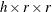) tables. For more information, see the section Tests for Equal Kappa Coefficients.

EQOR
ZELEN

includes Zelen’s exact test for equal odds ratios in the output data set. The EQOR option in the EXACT statement requests computation of this test, which is available for multiwaytables. For more information, see the section Zelen’s Exact Test for Equal Odds Ratios.

EQWKP

includes the test for equal weighted kappa coefficients in the output data set. The AGREE option in the TABLES statement requests computation of this test. The test for equal weighted kappas is available for multiway, square () tables where r > 2. For more information, see the section Tests for Equal Kappa Coefficients.

FISHER

includes Fisher’s exact test in the output data set. Fortables, the CHISQ option in the TABLES statement provides Fisher’s exact test. For tables larger than, the FISHER option in the EXACT statement provides Fisher’s exact test. For more information, see the section Fisher’s Exact Test.

GAMMA

includes the gamma statistic in the output data set. The MEASURES option in the TABLES statement requests computation of the gamma statistic. For more information, see the section Gamma.

GS
GAILSIMON

includes the Gail-Simon test for qualitative interaction in the output data set. The CMH(GAILSIMON) option in the TABLES statement requests computation of this test. For more information, see the section Gail-Simon Test for Qualitative Interactions.

JT

includes the Jonckheere-Terpstra test in the output data set. The JT option in the TABLES statement requests the Jonckheere-Terpstra test. For more information, see the section Jonckheere-Terpstra Test.

KAPPA

includes the simple kappa coefficient in the output data set. The AGREE option in the TABLES statement requests computation of kappa, which is available for square tables (where the number of rows equals the number of columns). For multiway square tables, the KAPPA option also includes the overall kappa coefficient in the output data set. For more information, see the sections Simple Kappa Coefficient and Overall Kappa Coefficient.

KENTB
TAUB

includes Kendall’s tau-b in the output data set. The MEASURES option in the TABLES statement requests computation of Kendall’s tau-b. For more information, see the section Kendall’s Tau-b.

LAMCR

includes the asymmetric lambda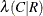in the output data set. The MEASURES option in the TABLES statement requests computation of lambda. For more information, see the section Lambda (Asymmetric).

LAMDAS

includes the symmetric lambda in the output data set. The MEASURES option in the TABLES statement requests computation of lambda. For more information, see the section Lambda (Symmetric).

LAMRC

includes the asymmetric lambda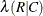in the output data set. The MEASURES option in the TABLES statement requests computation of lambda. For more information, see the section Lambda (Asymmetric).

LGOR

includes the logit estimate of the common odds ratio in the output data set. The CMH option in the TABLES statement requests computation of this statistic, which is available fortables. For more information, see the section Adjusted Odds Ratio and Relative Risk Estimates.

LGRRC1

includes the logit estimate of the common relative risk (column 1) in the output data set. The CMH option in the TABLES statement requests computation of this statistic, which is available fortables. For more information, see the section Adjusted Odds Ratio and Relative Risk Estimates.

LGRRC2

includes the logit estimate of the common relative risk (column 2) in the output data set. The CMH option in the TABLES statement requests computation of this statistic, which is available fortables. For more information, see the section Adjusted Odds Ratio and Relative Risk Estimates.

LRCHI

includes the likelihood ratio chi-square in the output data set. The CHISQ option in the TABLES statement requests computation of the likelihood ratio chi-square. For more information, see the section Likelihood Ratio Chi-Square Test.

MCNEM

includes McNemar’s test (fortables) in the output data set. The AGREE option in the TABLES statement requests computation of McNemar’s test. For more information, see the section McNemar’s Test.

MEASURES

includes the following measures of association in the output data set: gamma, Kendall’s tau-b, Stuart’s tau-c, Somers’, Somers’, Pearson and Spearman correlation coefficients, lambda (symmetric and asymmetric), and uncertainty coefficients (symmetric and asymmetric). Fortables, the MEASURES option also includes the odds ratio, column 1 relative risk, and column 2 relative risk. The MEASURES option in the TABLES statement requests computation of these statistics. For more information, see the section Measures of Association.

MHCHI

includes the Mantel-Haenszel chi-square in the output data set. The CHISQ option in the TABLES statement requests computation of the Mantel-Haenszel chi-square. For more information, see the section Mantel-Haenszel Chi-Square Test.

MHOR
COMOR

includes the Mantel-Haenszel estimate of the common odds ratio in the output data set. The CMH option in the TABLES statement requests computation of this statistic, which is available fortables. For more information, see the section Adjusted Odds Ratio and Relative Risk Estimates.

MHRRC1

includes the Mantel-Haenszel estimate of the common relative risk (column 1) in the output data set. The CMH option in the TABLES statement requests computation of this statistic, which is available fortables. For more information, see the section Adjusted Odds Ratio and Relative Risk Estimates.

MHRRC2

includes the Mantel-Haenszel estimate of the common relative risk (column 2) in the output data set. The CMH option in the TABLES statement requests computation of this statistic, which is available fortables. For more information, see the section Adjusted Odds Ratio and Relative Risk Estimates.

N

includes the number of nonmissing observations in the output data set.

NMISS

includes the number of missing observations in the output data set. For more information, see the section Missing Values.

OR
ODDSRATIO
RROR

includes the odds ratio (fortables) in the output data set. The MEASURES , OR , and RELRISK options in the TABLES statement request this statistic. For more information, see the section Odds Ratio.

PCHI

includes the Pearson chi-square in the output data set for two-way tables. For more information, see the section Pearson Chi-Square Test for Two-Way Tables. For one-way tables, the PCHI option includes the chi-square goodness-of-fit test in the output data set. For more information, see the section Chi-Square Test for One-Way Tables. The CHISQ option in the TABLES statement requests computation of these statistics.

If you specify the CHISQ(WARN=OUTPUT) option in the TABLES statement, the PCHI option also includes the variable WARN_PCHI in the output data set. This variable indicates the validity warning for the asymptotic Pearson chi-square test.

PCORR

includes the Pearson correlation coefficient in the output data set. The MEASURES option in the TABLES statement requests computation of the Pearson correlation. For more information, see the section Pearson Correlation Coefficient.

PHI

includes the phi coefficient in the output data set. The CHISQ option in the TABLES statement requests computation of the phi coefficient. For more information, see the section Phi Coefficient.

PLCORR

includes the polychoric correlation coefficient in the output data set. Fortables, this statistic is known as the tetrachoric correlation coefficient. The PLCORR option in the TABLES statement requests computation of the polychoric correlation. For more information, see the section Polychoric Correlation.

RDIF1

includes the column 1 risk difference (row 1 – row 2) in the output data set. The RISKDIFF option in the TABLES statement requests computation of risks and risk differences, which are available fortables. For more information, see the section Risks and Risk Differences.

RDIF2

includes the column 2 risk difference (row 1 – row 2) in the output data set. The RISKDIFF option in the TABLES statement requests computation of risks and risk differences, which are available fortables. For more information, see the section Risks and Risk Differences.

RELRISK

includes the column 1 and column 2 relative risks (fortables) in the output data set. The MEASURES and RELRISK options in the TABLES statement request these statistics. For more information, see the section Relative Risks.

RISKDIFF

includes risks (binomial proportions) and risk differences fortables in the output data set. These statistics include the row 1 risk, row 2 risk, total (overall) risk, and risk difference (row 1 – row 2) for column 1 and column 2. The RISKDIFF option in the TABLES statement requests computation of these statistics. For more information, see the section Risks and Risk Differences.

RISKDIFF1

includes column 1 risks (binomial proportions) and risk differences fortables in the output data set. These statistics include the row 1 risk, row 2 risk, total (overall) risk, and risk difference (row 1 – row 2). The RISKDIFF option in the TABLES statement requests computation of these statistics. For more information, see the section Risks and Risk Differences.

RISKDIFF2

includes column 2 risks (binomial proportions) and risk differences fortables in the output data set. These statistics include the row 1 risk, row 2 risk, total (overall) risk, and risk difference (row 1 – row 2). The RISKDIFF option in the TABLES statement requests computation of these statistics. For more information, see the section Risks and Risk Differences.

RRC1
RELRISK1

includes the column 1 relative risk in the output data set. The MEASURES and RELRISK options in the TABLES statement request relative risks, which are available fortables. For more information, see the section Odds Ratio and Relative Risks for 2 x 2 Tables.

RRC2
RELRISK2

includes the column 2 relative risk in the output data set. The MEASURES and RELRISK options in the TABLES statement request relative risks, which are available fortables. For more information, see the section Odds Ratio and Relative Risks for 2 x 2 Tables.

RSK1
RISK1

includes the overall column 1 risk in the output data set. The RISKDIFF option in the TABLES statement requests computation of risks and risk differences, which are available fortables. For more information, see the section Risks and Risk Differences.

RSK11
RISK11

includes the column 1 risk for row 1 in the output data set. The RISKDIFF option in the TABLES statement requests computation of risks and risk differences, which are available fortables. For more information, see the section Risks and Risk Differences.

RSK12
RISK12

includes the column 2 risk for row 1 in the output data set. The RISKDIFF option in the TABLES statement requests computation of risks and risk differences, which are available fortables. For more information, see the section Risks and Risk Differences.

RSK2
RISK2

includes the overall column 2 risk in the output data set. The RISKDIFF option in the TABLES statement requests computation of risks and risk differences. For more information, see the section Risks and Risk Differences.

RSK21
RISK21

includes the column 1 risk for row 2 in the output data set. The RISKDIFF option in the TABLES statement requests computation of risks and risk differences, which are available fortables. For more information, see the section Risks and Risk Differences.

RSK22
RISK22

includes the column 2 risk for row 2 in the output data set. The RISKDIFF option in the TABLES statement requests computation of risks and risk differences, which are available fortables. For more information, see the section Risks and Risk Differences.

SCORR

includes the Spearman correlation coefficient in the output data set. The MEASURES option in the TABLES statement requests computation of the Spearman correlation. For more information, see the section Spearman Rank Correlation Coefficient.

SMDCR

includes Somers’in the output data set. The MEASURES option in the TABLES statement requests computation of Somers’ D. For more information, see the section Somers’ D.

SMDRC

includes Somers’in the output data set. The MEASURES option in the TABLES statement requests computation of Somers’ D. For more information, see the section Somers’ D.

STUTC
TAUC

includes Stuart’s tau-c in the output data set. The MEASURES option in the TABLES statement requests computation of tau-c. For more information, see the section Stuart’s Tau-c.

TREND

includes the Cochran-Armitage test for trend in the output data set. The TREND option in the TABLES statement requests computation of the trend test. This test is available for tables of dimension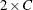or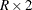. For more information, see the section Cochran-Armitage Test for Trend.

TSYMM
BOWKER

includes Bowker’s test of symmetry in the output data set. The AGREE option in the TABLES statement requests computation of Bowker’s test. For more information, see the section Bowker’s Test of Symmetry.

U

includes the uncertainty coefficient (symmetric) in the output data set. The MEASURES option in the TABLES statement requests computation of the uncertainty coefficient. For more information, see the section Uncertainty Coefficient (Symmetric).

UCR

includes the asymmetric uncertainty coefficient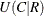in the output data set. The MEASURES option in the TABLES statement requests computation of the uncertainty coefficient. For more information, see the section Uncertainty Coefficients (Asymmetric).

URC

includes the asymmetric uncertainty coefficient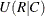in the output data set. The MEASURES option in the TABLES statement requests computation of the uncertainty coefficient. For more information, see the section Uncertainty Coefficients (Asymmetric).

WTKAP
WTKAPPA

includes the weighted kappa coefficient in the output data set. The AGREE option in the TABLES statement requests computation of weighted kappa, which is available for square tables larger than. For multiway tables, the WTKAP option also includes the overall weighted kappa coefficient in the output data set. For more information, see the sections Weighted Kappa Coefficient and Overall Kappa Coefficient.